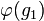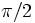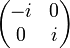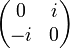# Character value need not determine similarity class of image under irreducible representation

## Statement

It is possible to have a finite group$G$ and an irreducible representation$\varphi$ of$G$ over a splitting field in characteristic zero (and hence also over$\mathbb{C}$) such that, if$\chi$ denotes the character of$\varphi$, we have elements$g_1, g_2 \in G$ satisfying:

•$\chi(g_1) = \chi(g_2)$, and
•$\varphi(g_1)$ and$\varphi(g_2)$ are not similar matrices, i.e., they are not conjugate in the general linear group to which the representation maps$G$. In particular, they may have different characteristic polynomials.

## Proof

### Example of the dihedral group

Further information: faithful irreducible representation of dihedral group:D8

Consider dihedral group:D8 (see also linear representation theory of dihedral group:D8) and its faithful irreducible two-dimensional representation.

The dihedral group of order eight has a two-dimensional irreducible representation, where the element$a$ acts as a rotation (by an angle of$\pi/2$), and the element$x$ acts as a reflection about the first axis. The matrices are:$a \mapsto \begin{pmatrix}0 & -1 \\ 1 & 0 \\\end{pmatrix}, \qquad x \mapsto \begin{pmatrix}1 & 0 \\ 0 & -1 \\\end{pmatrix}.$

This particular choice of matrices give a representation as orthogonal matrices, and in fact, the representation is as signed permutation matrices (i.e., it takes values in the signed symmetric group of degree two). Thus, it is also a monomial representation.

Below is a description of the matrices based on the above choice as well as another formulation involving complex unitary matrices:

Element Matrix (orthogonal/monomial/signed permutation matrices) Matrix as complex unitary Characteristic polynomial Minimal polynomial Trace, character value Determinant$e$$\begin{pmatrix} 1 & 0 \\ 0 & 1 \\\end{pmatrix}$$\begin{pmatrix} 1 & 0 \\ 0 & 1 \\\end{pmatrix}$$(t - 1)^2 = t^2 - 2t + 1$$t - 1$ 2 1$a$$\begin{pmatrix}0 & -1 \\ 1 & 0 \\\end{pmatrix}$$\begin{pmatrix}i & 0 \\ 0 & -i \\\end{pmatrix}$$t^2 + 1$$t^2 + 1$ 0 1$a^2$$\begin{pmatrix} -1 & 0 \\ 0 & -1 \\\end{pmatrix}$$\begin{pmatrix} -1 & 0 \\ 0 & -1 \\\end{pmatrix}$$(t + 1)^2 = t^2 + 2t + 1$$t + 1$ -2 1$a^3$$\begin{pmatrix}0 & 1 \\ -1 & 0 \\\end{pmatrix}$$\begin{pmatrix}-i & 0 \\ 0 & i \\\end{pmatrix}$$t^2 + 1$$t^2 + 1$ 0 1$x$$\begin{pmatrix}1 & 0 \\ 0 & -1 \\\end{pmatrix}$$\begin{pmatrix} 0 & 1 \\ 1 & 0 \\\end{pmatrix}$$t^2 - 1$$t^2 - 1$ 0 -1$ax$$\begin{pmatrix}0 & 1 \\ 1 & 0 \\\end{pmatrix}$$\begin{pmatrix} 0 & i \\ -i & 0 \\\end{pmatrix}$$t^2 - 1$$t^2 - 1$ 0 -1$a^2x$$\begin{pmatrix}-1 & 0 \\ 0 & 1 \\\end{pmatrix}$$\begin{pmatrix} 0 & -1 \\ -1 & 0 \\\end{pmatrix}$$t^2 - 1$$t^2 - 1$ 0 -1$a^3x$$\begin{pmatrix}0 & -1 \\ -1 & 0 \\\end{pmatrix}$$\begin{pmatrix} 0 & -i \\ i & 0 \\\end{pmatrix}$$t^2 - 1$$t^2 - 1$ 0 -1
Set of values used$\{ 0,1,-1 \}$$\{ 0,1,-1,i,-i \}$ -- --$\{ 2,-2,0 \}$$\{ 1,-1 \}$
Ring generated by values used (characteristic zero)$\mathbb{Z}$ -- ring of integers$\mathbb{Z}[i]$ -- ring of Gaussian integers -- --$\mathbb{Z}$ -- ring of integers$\mathbb{Z}$ -- ring of integers
Field generated by values used (characteristic zero)$\mathbb{Q}$ -- field of rational numbers$\mathbb{Q}(i) = \mathbb{Q}[t]/(t^2 + 1)$ -- --$\mathbb{Q}$ -- field of rational numbers$\mathbb{Q}$ -- field of rational numbers

We see from the table above that the elements$a$ and$x$ have the same character value, namely$0$, but their images have different characteristic polynomials (the characteristic polynomial of$a$ is$t^2 + 1$, that of$x$ is$t^2 - 1$) and are hence the images are not similar matrices.